# Abstract

A system is said to be "instantaneous" when for a given constant input an equilibrium output is obtained after a while. In the meantime, the output is changing from its initial value towards the equilibrium one. This is the transient period of the system and transients are important features of open-respirometry systems. During transients, one cannot compute the input amplitude directly from the output. The existing models (e.g., first or second order dynamics) cannot account for many of the features observed in real open-respirometry systems, such as time lag. Also, these models do not explain what should be expected when a system is speeded up or slowed down. The purpose of the present study was to develop a mechanistic approach to the dynamics of open-respirometry systems, employing basic thermodynamic concepts. It is demonstrated that all the main relevant features of the output dynamics are due to and can be adequately explained by a distribution of apparent velocities within the set of molecules travelling along the system. The importance of the rate at which the molecules leave the sensor is explored for the first time. The study approaches the difference in calibrating a system with a continuous input and with a "unit impulse": the former truly reveals the dynamics of the system while the latter represents the first derivative (in time) of the former and, thus, cannot adequately be employed in the apparent time-constant determination. Also, we demonstrate why the apparent order of the output changes with volume or flow.

open-respirometry systems; thermodynamic model

Braz J Med Biol Res, August 2000, Volume 33(8) 967-982

Further analysis of open-respirometry systems: an a-compartmental mechanistic approach

J.G. Chaui-Berlinck and J.E.P.W. Bicudo

Departamento de Fisiologia, Instituto de Biociências, Universidade de São Paulo, São Paulo, SP, Brasil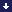ReferencesCorrespondence

Abstract

A system is said to be "instantaneous" when for a given constant input an equilibrium output is obtained after a while. In the meantime, the output is changing from its initial value towards the equilibrium one. This is the transient period of the system and transients are important features of open-respirometry systems. During transients, one cannot compute the input amplitude directly from the output. The existing models (e.g., first or second order dynamics) cannot account for many of the features observed in real open-respirometry systems, such as time lag. Also, these models do not explain what should be expected when a system is speeded up or slowed down. The purpose of the present study was to develop a mechanistic approach to the dynamics of open-respirometry systems, employing basic thermodynamic concepts. It is demonstrated that all the main relevant features of the output dynamics are due to and can be adequately explained by a distribution of apparent velocities within the set of molecules travelling along the system. The importance of the rate at which the molecules leave the sensor is explored for the first time. The study approaches the difference in calibrating a system with a continuous input and with a "unit impulse": the former truly reveals the dynamics of the system while the latter represents the first derivative (in time) of the former and, thus, cannot adequately be employed in the apparent time-constant determination. Also, we demonstrate why the apparent order of the output changes with volume or flow.

Key words: open-respirometry systems, thermodynamic model

# Introduction

The dynamics of a respirometry system is an essential feature of such a system and investigators must be fully familiarized with it before experiments begin. This is due to the fact that respirometry systems have transients, i.e., periods of time during which the output amplitude (the signal) of the system is below the amplitude of the input. Therefore, during transients, one cannot compute the amplitude of the input (e.g., oxygen consumption, carbon dioxide production, etc.) directly from the output. Two general solutions are possible. The first is to work with very fast systems which behave as zero-order systems (in which transients are not detected). However, in most cases this is not usually a feasible task for the well-known limits of open-respirometry systems (i.e., flow, volume, the biological system under investigation, etc.). The second solution is to transform the output into instantaneous readings by some mathematical procedure (1), i.e., to obtain output amplitudes linearly related to the input amplitudes. Three branches of such solutions are found in the literature: a) single-chamber first-order models (2-10), b) two-chamber second-order models (11), and c) unknown number of chambers free-of-order models (12,13). The first-order models analyze the output as coming from a single volume of dilution, and the Z-transformation of the output is the mathematical procedure used to obtain instantaneous readings (4) even though adding the output to its first derivative in relation to time is another possibility (7-9). Free-of-order models employ deconvolution of the output by a transfer function obtained during calibration procedures. The putative number of volumes diluting the input is irrelevant to this transformation, and the procedure is simply instrumental in this respect.

Frappell et al. (11) proposed a two-chamber model that resulted in a second-order solution. They stated the superiority of their model in relation to first-order ones based on the fact that the second-order function profile is much more similar to empirical output profiles than are first-order function profiles. Several flaws can be detected in their model, and we list them in Appendix A. One of these flaws (perhaps the most relevant one) is the statement that the profile of the output will approach a first-order dynamics if the first chamber is large in relation to the second one (or vice-versa). Empirically, one can demonstrate that the larger the volume of a chamber the less the output is close to a first-order model (see Figure 5A). This phenomenon is not related to an a priori predetermined number of chambers counted from an anthropocentric point of view.

Let us state the problem in another way. Consider a given system (Footnote 1) Syst1 with an output that apparently obeys a given order (e.g., N order). Then, increasing the volume of such a system or decreasing the convective flow through it (i.e., slowing down the system) would result in a) a system (Syst2) that is of the same N order as Syst1 but with different values for the time constants that describe it, or b) a change in the order of Syst2 in relation to the apparent N order describing Syst1. Mutatis mutandis, the question holds true for the opposite, i.e., when a system is speeded up. Empirically, we already know that a change in the order of the apparent function describing the output will occur. However, none of the present models explains why and how this happens to be so. These models also cannot account for the so-called "time lag" (i.e., a finite amount of time between the input taking place and some alteration occurring in the sensor cell) (Footnote 2). All these problems are present in such models because the latter are not truly describing the mechanics of the systems. Instead, they are fitting a pre-set of differential equations to the observed output.

In the present study we developed an approach to open-respirometry system dynamics employing basic concepts of thermodynamics and statistical mechanics. This approach allows an unequivocal understanding of the principles underlying the relationship between volume and flow in open-respirometry systems, thereby clearly explaining why and how there is a change in the apparent order of the output when a system is speeded up or slowed down. We also explore the role of the sensor cell itself in the system and the difference between calibrating an open-respirometry system (i.e., how to obtain its dynamics) by a continuous input and by a unit impulse.

# Methods and Results

The basic experimental set-up

Appendix B contains a list of symbols and definitions. We describe here the main features of open-respirometry systems. These systems consist of tubes connected to each other in series. The system is said to initiate at the L0 point, i.e., the point where the system starts physically (before L0 is the outside environment). The series of connected tubes reaches the entrance of the sensor cell, S, at LS. The sensor cell has a given volume and ends at a point called LS+Ds. Finally, the system ends at a point called Lf: beyond Lf there is, again, the outside environment. There is a convective flow,, of ambient gas through the system from L0 to Lf.determines a sense of convective flow within the system, defined as the x+ (x positive) sense. The input into the system occurs at a point called L0IN. For practical reasons we will simply state that L0IN>L0, i.e., the input occurs beyond the entrance to the system, in the x+ sense. The connected tubes consist of a volume which, from the "output point of view", extends from L0IN to LS+Ds (not just to LS) as we will demonstrate below. The gas molecules of the input flow through the system from L0IN. Figure 1 illustrates the main features of open-respirometry systems. For the sake of simplicity, but without loss of generality, the following constraints are part of this approach: a) temperature is constant; b) the cross-section of the connected tubes is constant; c) the sensor cell has the same cross-section as the other tubes; d) the external environment acts as an infinite pool and sink; e) all gas molecules have the same mass and diameter, and collisions are elastic; f) the system is in a steady-state of pressure, i.e., the transients of the convective flow have finished, and g) there is no input loss (this is why L0IN>L0) and turbulence will not be accounted for in the approach.

Main symbols and definitions

If all molecules had the same velocity in the x+ sense of the system the output of any given input would look like a square pulse. However, in real systems, this is not the case, because the input molecules do not have all the same velocity, as will be demonstrated below.

Real velocities of the particles - total and the X component

At any given temperature (greater than 0 K) the distribution of velocities in a population of gas molecules follows a Maxwell distribution (14):

(Equation 1)where M is the molar mass of the molecules, R is the gas constant, T is temperature and v is the specified velocity of a population f of molecules (Figure 2A). At any Lj point along the system (L0<Lj<Lf) the profile of velocities described by equation 1 is expected to be found. Consider now a step change in the input that occurs at t0 (t0 is the exact moment when a transition in the amplitude of a former constant input occurs). This new input is composed of a set of molecules whose velocity profile is described by equation 1 as well, and this set of molecules shows this profile at any observation time (given that we are considering a conservative system, item (a) above). However, this does not mean that each molecule in the set is locked in a fixed velocity. On the contrary, each time a molecule collides its velocity changes. Thus, each molecule tends to reach a mean velocity due to collisions. Over time, the set of these molecules can be described as having a mean velocity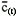(the mean velocity of the Maxwell distribution: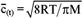although the profile of the set (given by equation 1) is the same at any particular time. This mean velocity has a standard deviation,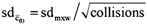, where sdmxw is the standard deviation of the original (Maxwell) distribution divided by the square root of the number of collisions of the particle from t0 to t (the result for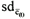is simply the Law of Large Numbers or central limit theorem (15)). Due to the huge number of collisions a particle suffers within short periods of time (see below) we will simply useandinstead ofand, respectively. Therefore, a gaussian curve adequately describes the velocity profile of the particles in a given initial set:

•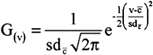(Equation 2)
• We insist in the difference between equations 1 and 2: the profile of velocities is the one described by equation 1 at any given moment of observation of the set, and the gaussian curve of equation 2 is the net result of the changes in velocity of the particles along time. Given that each molecule in a gas phase under ordinary conditions collides about 109 times per second, the mean velocity of each particle tends to be determined in the very first second after the input takes place. Figure 2 illustrates these points.

Thus, it was shown that the total velocity, v, tends to reach a mean value for each particle as time goes by. This total velocity is the vector composed by the three orthogonal components of velocity:

•(Equation 3)
• These orthogonal components are independent of each other, i.e., changes in one of them do not imply changes in the other ones (as long as the temperature is constant and, therefore, the total kinetic energy of the initial set is constant (14)). We are concerned with the travelling rate in the x direction, i.e., with the speed of the particles going from L0IN to LS+Ds. The distribution of velocities on a given axis (e.g., x) is:

•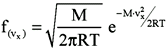(Equation 4)
• With time, each molecule in the initial set attains a mean velocity on such an axis, and, as seen before, the set can be described as having a mean velocity on the axis () and a standard deviation of this mean ():

•(Equation 5)
• It should be noted that equation 5 describes the mean velocity in the x direction (or any other orthogonal axis), but is blind to the sense of the motion (i.e., it represents both the positive and the negative components in the given direction). We will now consider what happens when convective flow is present.

Apparent velocities of the particles

Gas particles are in a constant rocking motion, coming and going all the time. They collide with each other and, at such collisions, they can change the sense of their motion (e.g., from a positive x sense to a negative one). When convective flow is imposed on the system, there is nothing that really pushes or pulls each particle. Instead, there is a higher probability that a particle once moving in the positive sense of the flow (as defined above) will continue in such a sense longer than when it is moving in the opposite one. Given that changing in the sense of the motion only occurs when particles having opposite senses collide, we can write the difference in probability that a particle would change sense at a given point Lj of the system as:

•(Equation 6)
• where z stands for the collision frequency that a particle experiences at Lj when travelling in the positive (z+) and in the negative (z-) sense of the x axis. Thus, when collision frequency is the same in both senses (no convective flow is present) L = 0, i.e., only the probability of diffusion affects the movement of particles from one point to another. A good approximation for L (as we justify in Appendix C) is:

The proportion of time that a particle spends travelling in the positive sense of the flow

The frequency of collisions (z) of a particle is (14):

•(Equation C1)
• where s is the collision cross-section of the molecules, N is the density of particles in a given volume and

•(Equation C2)
• where = 2.

•(Equation C3)
• as stated above, a particle will change its sense of motion when it collides with a particle coming from the opposite sense. Thus, let us define a function L that measures (or estimates) the proportion of displacement that a particle makes in a specified sense in relation to the opposite displacement. Therefore, L ends up estimating the amount of time a particle spends travelling in a given sense in relation to the amount of time travelling in the opposite sense.

Convective flow is generated either by compression or by suction. When compression is the causal factor there is an increase in the collision frequency in the negative sense of the flow (i.e., upstream). On the other hand, when suction is the causal factor, there is a decrease in the collision frequency in the positive sense of the flow (i.e., downstream). Both the increase and decrease just cited are related to the velocity of the moving wall of a piston, Dw. Consider, for example, a particle moving towards the wall of the piston (in a suction set-up) with velocity vx and at a distance d from the wall. If the wall is not moving, after a time of d/vx the particle is expected to be found colliding with the wall. If the wall is moving with a speed of Dw (Dw<<vx), after a time of d/vx the particle would not have reached the wall, which is Dw.d/vx units of length ahead. Therefore, a second particle expected to collide with the first one (when the latter would be returning after an elastic shock with the still wall) experiences a decrease in the collision frequency, proportional to the difference vx - Dw when the wall is moving. Therefore, we will write the L function for suction and compression as, respectively:

•(Equation C4a)
•(Equation C4b)
• Notice that L is independent of the Lj position in the system where it is being evaluated. Also, notice that Dw<<Dw/

•(Equation C5)
• gives a good estimate of the proportion of time that particles spend travelling in the positive sense of convective flow.

•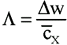(Equation 7)
• where Dw is the absolute value of the velocity of the moving piston causing convective flow andis the mean velocity of the particles in the x direction. Notice that L is independent of the Lj point of the system. The apparent velocity (in the x direction) of a particle will be the product of its mean velocity and L. Therefore, the whole set has a mean apparent velocity indicated by the following equation:

•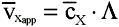(Equation 8)
• A note on variance: many factors were not taken into account in this study. Some of them are turbulence, inhomogeneities of the gas medium, changes in the geometry of the tubing, and the variance in L. Analysis of such factors is beyond the scope of the present study. However, it is very important to note that these added variances make a considerable contribution to the final spreading of velocities around the mean value.

We will not propagate error and the standard deviation of the above apparent mean velocity will be simply described as

.L, where the superscript + indicates that added components of variance should be included. Normality is preserved (Footnote 3) and the set of particles can be adequately described by a gaussian function as:

(Equation 9)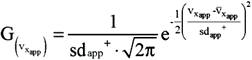Equation 9 is the foundation of this study. It tells us that any given initial set of particles will attain a mean apparent velocity on the axis of the convective flow imposed on the system, and that particles of such a set will have apparent velocities normally distributed around the mean velocity of the set. This obviously explains, unequivocally, the existence of the time lag between an input and the beginning of the corresponding output: it is the length of time that the faster particles of the input take to travel from L0IN to LS (even though a gaussian function ranges from -¥ to +¥, for practical purposes the entire population can be considered to lie between -3sd and +3sd around the mean, so there will exist a minimum time required for the faster particles to travel). In the next section we will explore the output dynamics that results from equation 9.

Output dynamics of open-respirometry systems

The input. Most of the arguments of the functions will be omitted for the sake of simplicity (e.g., G instead of). Let us consider a set A of gas molecules as an unitary amplitude input into the system. Therefore, such a set has particles which have velocities distributed according to function G (equation 9). Obviously, set A has a total number of molecules corresponding to the integral H of G (because the velocities in this case are equal to or greater than zero, the integral will be evaluated only in the positive range):

(Equation 10)The total amount of molecules in a continuous input into the system at time t is the integral of A in relation to time, from t0 = 0 to t:

(Equation 11)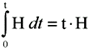The output. Let us define a function E(t) representing the state of the sensor at time t. The state of a sensor is a function (linear, in general) of the occupancy level of the sensor by the subject of measurement of that sensor. The entry rate into the sensor is the amount of molecules (subject of measurement) that cross the entrance to the sensor cell at LS. The exit rate is the amount of molecules leaving the sensor cell at LS+Ds:

(Equation 12)Therefore, the output dynamics of an open-respirometry system, equivalent to the state E(t) of the sensor, is only a function of the velocities of the particles of the input travelling a linear distance to reach the entrance and then the exit of the sensor. The apparent mixing or dilutional features of these systems are just the result from the difference in the time of arrival, at the sensor, of the particles of a given input set (see below). This was never taken into account before. By examining equation 12 we can see that when

i.e., roughly speaking, when the exit rate equals the entry rate,and so, E(t) = constant. At that time, the output stabilizes at a level, and such a level is the same as the amount of molecules coming from a corresponding time interval at the input (see equation 10). In other words, the output becomes linearly related to the input.

Another point that was also never appreciated before is that the leaving rate of the gas molecules from the sensor is crucial to the output dynamics. To stress such a point, consider a very long sensor (in the x direction), in a way that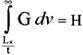at a time t wheni.e., the entry rate attains a stable value at a time when the exit rate still equals zero. Roughly speaking again, the slowest subset of molecules enters the sensor while the fastest subset has not reached the exit of the measuring device. Under these conditions, the output becomes a linear function of time (from equation 11, E(t) = t.H) (Footnote 4)! This would be impossible to understand using other current models interpreting the output of open-respirometry systems. Even when the sensor is not that long some linear component can be present, arising from the slower subset(s) of molecules.

Particular solution for a small measuring device. Let us consider a very short sensor (in relation to the convective flow employed), where LS@ LS+Ds, and let us state without proof that this happens to be the most usual finding in open-respirometry systems. Thereby, the integral of equation 12 becomes (see Appendix D):

Justification of the use of equation 13 to describe the output of a "very short sensor"

The integration of equation 12 over time has no analytic solution. However, the problem of the length of the sensor can be demonstrated in another way. Let us define a as a subset of A composed of a class of molecules that has the same apparent velocity in the x direction, say va. Thus, in A, a contains G(va) particles. The constant input constituted by a begins at t0. Thus, a time lag tm1a until the initial a molecules reach the entrance to the sensor will exist:

•(Equation D1)
• The same molecules will reach the outlet boundary of the sensor at a time tm2a:

•(Equation D2)
• Therefore, for the homogeneous input a:

•(Equation D3)
• where en(t) and ex(t) stand for the entry and exit rates, respectively. By integrating both rates of equation D3 in relation to time we obtain the state of the sensor:

•(Equation D4)
• Notice that for tm1a£t£tm2a a linear increase with time occurs in the occupancy level of the sensor by molecules of the specified class. For t>tm2a the occupancy level of the sensor by the a class attains a plateau. Such a plateau value is of the general form Ti.G(vi) where Ti = tm2i - tm1i . As can be seen, each class of molecules will have a different T due to the different time each class takes to cross the sensor cell. The important point to be noted is that linearity with time should be expected in the output while t<tm2i.

Let us define a very short sensor as one for which Tslowest®0, i.e., the time the slowest subset of A takes to cross the sensor can be neglected on practical grounds. Note that this is not a limit, because LS+Ds - LS is measurable. Obviously, Ti for all the other classes can be neglected as well. Therefore, we may consider that each class of velocity of A is represented at the sensor proportionally to the other classes, and such a proportionality is given only by each G(vi) (because the different Tis are overlooked). Thus, under the assumption of a very short sensor, to obtain E(t) integrating equation 12 requires integrating G(vi), and this is H, as found in equation 13.

(Equation 13)Equations 12 and 13 are useful to approximate the real output. Thus, the derivative of the output of the system is the distribution function of the apparent velocities of the particles (equation 9), and the output itself is the integral of that gaussian function. Figure 3 illustrates numerical solutions of equation 12 and equation 13 for constant input in systems with different volumes (notice that volume is the linear distance from L0IN to LS) under the same convective flow (apparent velocity of the particles). Notice how the profiles of the output suggest the existence of dilutional spaces (volumes) dictating the dynamics of the systems. Increasing the volume of the system (i.e., increasing the linear distance from L0IN to LS) appears to increase the number of "volumes" diluting the output, and this will be discussed below. However, before approaching this subject we will examine another common calibration procedure, the "unit impulse". In this technique, a set of particles A makes up a unique input which occurs within an interval of "zero" units of time. Therefore, as in equation 10,Thus, the output, when integrated over time, results in H, and as a consequence (Footnote 5):

•(Equation 14)
• where the superscript ui stands for "unit impulse". Notice that the output of a unit impulse (equation 14) corresponds to the first derivative in relation to time of a continuous input (equation 12). When the time constants of a given system are determined using an exponential decay model (as the current models) there would be little distortion in such constants, because the exponents in those decays are the same in both the integral and its derivative. However, authors overlook the ascending portion of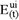(Footnote 6) (see Figure 3A and next section) and look only at the descending ("exponential decay") part of the curve. Time constants are then adjusted to this descending portion of the curve. This can lead to serious distortions in the reconstituted experimental inputs.

Change in output "order" with the slowing down or the speeding up of open-respirometry systems

This section is devoted to the problem stated in the Introduction of this study: increasing the volume of a Syst1 system or decreasing the convective flow through it (i.e., slowing down the system) would result in a Syst2 system that is of the same order as Syst1 but with different values of the time constants describing it, or would there be a change in the order of Syst2 in relation to the apparent order describing Syst1? We will demonstrate that the latter alternative is the case. Firstly, we will approach the causes leading to a change in the apparent order of the output dynamics occurring in a system that is being described by a single time constant, i.e., a putative first-order output. Then, we will extend the conclusion to any given apparent order of open-respirometry systems. Throughout the analysis it is necessary to bear in mind that we are considering functions which tend to an asymptotic value as time goes by. Thus, any pair of vectors representing such functions will tend to have norm®0 as t®¥ simply because they both tend to stabilize at a certain value. Therefore, in the following reasoning, we are concerned only with the period of time within which transients are present. In a general form: 0 = t0£t£T, where t0 = 0 is the time when the output begins to be detected and T is a later time when the total amplitude of the output is attained, on practical grounds. Note that t0 in this section has a different meaning from that used in the preceding ones, because it is not the initial time at which the input takes place.

Consider a Syst1 system where ||Y1(t). H1(t)|| = e1 (where ||w1.w2|| is the norm between two vectors w1 andw2), and one tends to accept function Y as a good approximation to H within an e1 error. The Y function has the general form shown below (equation 15).

Proposition: given a system with output H1(t) (H(t) is found in equation 13) for which an approximation Y1(t) @ H1(t) (within an error e1) is considered valid, the progressive increase of LS (Footnote 7) or decrease ofwill always result in ||Y2(t).H2(t)||>e1 with H2(t)<<Y2(t) for all t<tcross (to be defined below). That is, at the beginning of the output, the approximating (new) function Y(t) has values progressively greater than those of the real H(t) function as a given system is slowed down.

Demonstration. Let functions f1 and f2 be:

(Equation 15)where l is the inverse of a time constant. One intends to minimize(let this function be f3) by varying l. The traditional method to do so is to find the value of l for which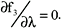Resolving the square and taking the first derivative of f3 in relation to l, one obtains the following result:

(Equation 16)Therefore, because there are no squares in either term of equation 16, it becomes implicit that part of the values of Y(t) must be higher than the corresponding H(t) and another part must be lower for the sum to result in zero (unless Y(t)º H(t), see below). If Y(t)º H(t), it follows that, but:

•(Equation 17)
• invalidating the putative Y(t)º H(t).

The crossing time of G(t) and l.e-l.t , tcross¶, is:

(Equation 18)The initial conditions are:

(Equation 19)So:

•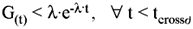(Equation 20)
• Let us define tcross (tcross>0) as the time when Y(t) and H(t) cross each other after their initial crossing at t0 = 0 (equation 19). It follows that Y(t)>H(t) for all t<tcross, that is, the solution of the minimization (equation 16) implies that the initial part of the approximating function Y(t) has higher values than the real function H(t). Because tcross¶ is directly proportional to LS (equation 18) and equation 16 is a minimization, then Y2(t) (for all t<tcross) is progressively higher than H2(t) as LS is progressively increased (and the same is valid for decreasing), irrespective of wether the computed value l2 (Footnote 8) satisfies equation 16 or any other metric minimization procedure. In other words, as an initial system 1 in which it is accepted that Y1(t) @ H1(t) is slowed down and becomes system 2, the approximating function Y2(t) has greater values than the real function H2(t) from t = t0 until their crossing at a later time (tcross). The vectors representing the functions fall progressively apart. At the beginning of the output, the error e2 is greater than the formerly accepted e1 for the same period. This phenomenon, under compartmental analysis, emerges as the necessity of adding more "dilutional volumes" to a sum of exponentials (i.e., more time constants in order to "slow down" the initial part of the approximate function).

Consider any output H(t) being described (by approximation) by a function Y(t) in the general form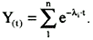Any li represents a relationship between a putative "dilutional volume" and flow. Thus, the above reasoning extends to any number of time constants employed to describe the output of a given system. This reasoning demonstrates the obligatory change in the apparent order of the system output, and, therefore, the impossibility of a given order be the same as open-respirometry systems are speeded up or slowed down. Note that the definition of volume (LS) is not anthropocentric, i.e., we are not "counting chambers". Instead, LS is a continuum measure, blind to "chamber" definitions.

Some experiments were done in order to illustrate the points demonstrated above: a) the change in the apparent order of output as volume and flow are changed, and b) that this is not linked to the number of "chambers" from an anthropocentric viewpoint. Figure 4 shows the system employed. Note that there is a single chamber whose volume can be increased or decreased on the parallel axis to the convective flow, which in turn can be increased or decreased as well. Figure 5 presents the empirical results obtained by changing volume or flow in the system described above. Also, numerical evaluations of integrals of the general form of equation 13 are shown. Notice the fine adjustment between empirical data and the predicted functions (see legend).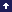Transients are important features of open-respirometry systems. Whether they are detected or not in real data acquisition procedures is a question that has to be known beforehand by the researcher. In cases where transients are detected, and the input is to be evaluated during such transients, the dynamics of the output is a major problem. In this study we employed basic concepts of thermodynamics and statistical mechanics to develop an approach to the problem of the output dynamics of open-respirometry systems.

The main result of this mechanistic approach is that molecules of the input travel a linear distance from the place where such an input takes place to the sensor, with an apparent velocity normally distributed around a mean value (function G in equation 9). All the important features of the output dynamics are determined by this travelling rate of the molecules which end up reaching and leaving the sensor cell at different times. This is the observed dynamics. Once this is recognized and quantified, time lag (the time between the beginning of input and the time where something begins to be detected at the sensor) becomes easily and unequivocally explained. Other current models cannot account for such an explanation. This study also revealed that the rate at which the molecules leave the sensor cell is an important part of the observed dynamics, a fact never considered before. This leads to a particular solution to the output for "very short sensors" (equation 13). Unless such a particular solution can be applied to a real experimental set-up, the presence of linear components in the output should be expected, and the current exponential approaches would be inherently inappropriate.

By applying the concepts developed, it was demonstrated that exponential decays are only functions approximating the real output one. The difference between calibrating the system with a continuous input and a "unit impulse" is stressed when one recognizes that the latter is the first derivative of the former. The resulting output is, therefore, the velocity distribution function G instead of its integral H. The distribution function G is composed of an ascending portion that cannot be simply ignored when computing the time constants of the approximating function to the output.

Finally, why and how the putative order of a system will change as the system is slowed down or speeded up is also presented. The main point is that at the beginning of the detected signal the approximate exponential function tends to have values progressively greater than the real output function as a system is slowed down. Therefore, more time constants need to be included in the approximate function for an adequate description of the output. This last result implies that neither a first-order model nor a second-order one can be taken for granted based on a system formerly evaluated or on the number of chambers counted from an anthropocentric point of view.

A very important point to be noted is that the present study was not intended to develop a new kind of signal reconstitution procedure for transients in open-respirometry systems. Therefore, under clinical conditions or in any other situations, this model should not be directly applied in order to obtain the input. However, this model opens the way to new approaches to the recovery of the input based on this mechanistic view of the process.

Discussion

# Acknowledgments

We would like to thank Dr. R. Ranveaud, Dr. M.V. Baldo and two anonymous reviewers for their criticisms, which improved the quantification analysis presented here.

Footnotes

Footnote 1) This means all the chambers and sub-chambers, tubing, sensor cells, the convective flow, the absorbents, etc.

Footnote 2) Authors simply take for granted the existence of the time lag, without any further consideration.

Footnote 3) And this is true because these errors are normally distributed as well.

Footnote 4) Until molecules reach the outlet boundary and thus begin to leave the sensor. Note that this linearity is completely different from a linear relationship between the input and the output, as discussed above. This is a linear increase of the output in relation to time.

Footnote 5) We are still working with the constraint of a very short sensor.

Footnote 6) Do they consider that the peak of the curve corresponds to the total amplitude of the unit impulse input itself?

Footnote 7) The linear distance from the input to the entrance of a very short sensor (see above) directly related to the volume of the system.

Footnote 8) The time constant of Syst2, a slowed down system in relation to Syst1.

• Correspondence
• Address for correspondence: J.G. Chaui-Berlinck, Departamento de Fisiologia, Instituto de Biociências, USP, 05508-900 São Paulo, SP, Brasil. Fax: +55-11-818-7422. E-mail: jgcb@usp.br

Research supported by a FAPESP grant to J.E.P.W. Bicudo. Part of a PhD thesis presented by J.G. Chaui-Berlinck to the Departamento de Fisiologia, Instituto de Biociências, Universidade de São Paulo, and supported by a CAPES fellowship. Received June 23, 1999. Accepted March 10, 2000.

• 1. Walliser B (1977). Systčmes et Modčles: Introduction Critique ŕ L'Analyse de Systčmes Éditions du Seuil, Paris.
• 2. Depocas F & Hart S (1957). Use of the Pauling oxygen analyzer for measurement of oxygen consumption of animals in open-circuit systems and in a short-lag, closed-circuit apparatus. Journal of Applied Physiology, 10: 388-392.
• 3. Tucker VA (1965). The relation between the torpor cycle and heat exchange in the California pocket mouse, Perognathus californicus. Journal of Cellular and Comparative Physiology, 65: 405-414.
• 4. Bartholomew GA, Vleck D & Vleck CM (1981). Instantaneous measurements of oxygen consumption during pre-flight warm-up and post-flight cooling in sphingid and saturniid moths. Journal of Experimental Biology, 90: 17-32.
• 5. Ravussin E, Lillioja S, Anderson TE, Christin L & Bogardus C (1986). Determinants of 24-hour energy expenditure in man. Journal of Clinical Investigation, 78: 1568-1578.
• 6. Pennock BE & Donahoe M (1993). Indirect calorimetry with a hood: flow requirements, accuracy, and minute ventilation measurement. Journal of Applied Physiology, 74: 485-491.
• 7. Sun M, Reed G & Hill JO (1994). Modification of a whole room indirect calorimeter for measurement of rapid changes in energy expenditure. Journal of Applied Physiology, 76: 2686-2691.
• 8. Heymsfield SB, Allison DB, Pi-Sunyer FX & Sun Y (1994). Columbia respiratory-chamber indirect calorimeter: a new approach to air-flow modelling. Medical and Biological Engineering and Computing, 32: 406-410.
• 9. Henning B, Löfgren R & Sjöström L (1996). Chamber for indirect calorimetry with improved transient response. Medical and Biological Engineering and Computing, 34: 207-212.
• 10. Seale JL & Rumpler WV (1997). Synchronous direct gradient layer and indirect room calorimetry. Journal of Applied Physiology, 83: 1775-1781.
• 11. Frappell PB, Blevin HA & Baudinette RV (1989). Understanding respirometry chambers: what goes in must come out. Journal of Theoretical Biology, 138: 479-494.
• 12. Kaufmann, R, Forstner H & Wieser W (1989). Respirometry - methods and approaches. In: Bridges CR & Butler PJ (Editors), Techniques in Comparative Respiratory Physiology Cambridge University Press, Cambridge, UK.
• 13. Ferrannini E (1992). Equations and assumptions of indirect calorimetry: some special problems. In: Kinney JM & Tucker HN (Editors), Energy Metabolism: Tissue Determinants and Cellular Corollaries Raven Press Ltd., New York.
• 14. Atkins PW (1998). Physical Chemistry 6th edn. W.H. Freeman and Company, New York.
• 15. Sokal RR & Rohlf FJ (1997). Biometry 3rd edn. W.H. Freeman and Company, New York.

# Appendix B: mean velocity of the Maxwell distribution: mean velocity of the particles in the x direction: mean relative velocity (total): mean relative velocity of the particles on the x axis: mean apparent velocity of a set of molecules in the: mean apparent velocity of a particle on the positive: standard deviation of the mean velocity: standard deviation of the mean velocity in the x direction: standard deviation of the mean apparent velocity in the
•: mean velocity of the Maxwell distribution
•: mean velocity of the particles in the x direction
•: mean relative velocity (total)
•: mean relative velocity of the particles on the x axis
•: mean apparent velocity of a set of molecules in the
positive sense of the x direction
•: mean apparent velocity of a particle on the positive
sense of the x direction
•: standard deviation of the mean velocity
•: standard deviation of the mean velocity in the x direction
•: standard deviation of the mean apparent velocity in the
positive sense of the x direction (with added components of
variance also taken into account)
• Dw: the velocity of a piston wall causing convective flow

E(t): the state of a sensor at time t

G: the gaussian function of velocity distribution

H: the integral of G

l: a time constant

L: difference in probability that a particle would change

sense in the sense of the convective flow

L0: the entry of an open-respirometry system

L0IN: the place where input takes place in an

open-respirometry system

Lf: the exit of an open-respirometry system

Lj: any point between L0IN and LS

LS: the entry of the sensor

LS+Ds: the exit of the sensor

t0: time when a new input begins (or the beginning of

the output corrected for the time lag, as in section

"Change in output "order" with the slowing down or the

speeding up of open-respirometry systems"

Y(t): an exponential function approximating the output of

an open-respirometry system

z: collision frequency

# Appendix D

. During the travel, the gas particles pass through a sensor that begins at LS and ends at LS+Ds. The entry of the system is identified at L0, a point before L0IN. (Equation 2) (Equation 3) (Equation 4) (Equation 5) (Equation 6) (Equation C1) (Equation C2) (Equation C3) (Equation C4a) (Equation C4b) (Equation C5) (Equation 7) (Equation 8) (Equation D1) (Equation D2) (Equation D3) (Equation D4) (Equation 14) and E(t) (equations 12 and 13, respectively). Parameters were (in arbitrary units): mean velocity = 200, standard deviation = 50. The solutions were computed for 4 different putative volumes of the system, i.e., 4 different distances from L0IN to LS. (a = 100, b = 250, c = 500, d = 1000). The sensor volume was fixed as 5 arbitrary linear units (i.e., LS+Ds - LS = 5). A, Solutions of (t) for a unit impulse calibrating input (see text). There is an ascending part of the curves that is truly part of the output, and cannot be slight when "time constants" are computed for unit impulses (see text). B, Solutions of E(t). The outputs of the different volumes (a, b, c, and d) are shown, without time lag correction. Using the same time scale of data acquisition, an increase in the order of the system will appear to occur from a to d and, thus, more time constants should be added (see text). (Equation 17) (Equation 20) Correspondence

# Publication Dates

• Publication in this collection
31 July 2000
• Date of issue
Aug 2000